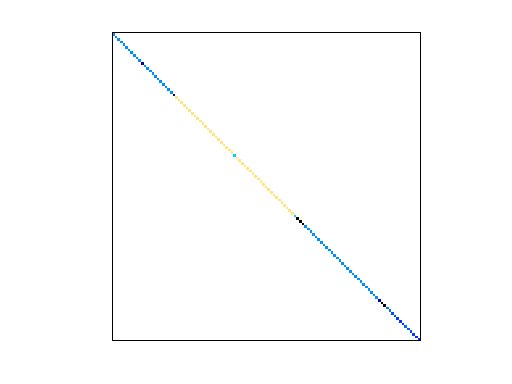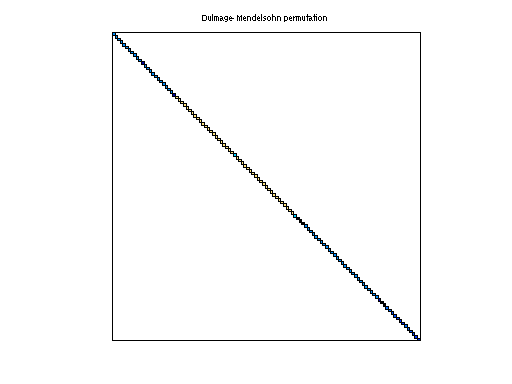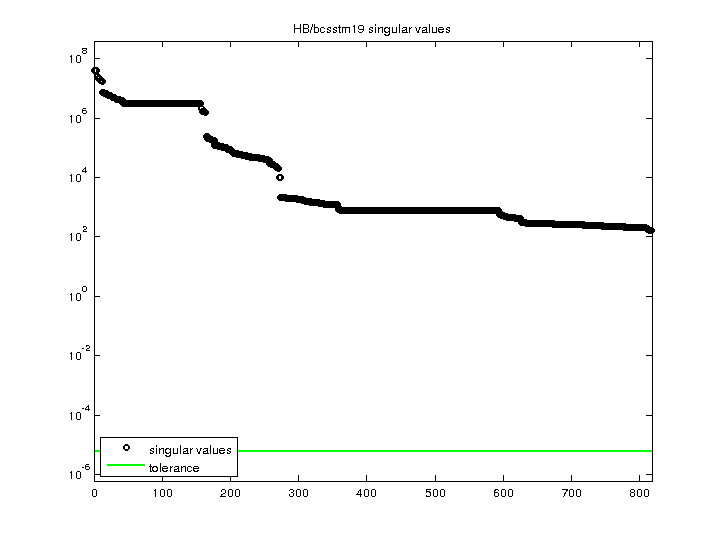Matrix: HB/bcsstm19

Description: SYMMETRIC MASS MATRIX - PART OF A SUSPENSION BRIDGE(undirected graph drawing)• Matrix group: HB
• download as a MATLAB mat-file, file size: 4 KB. Use UFget(69) or UFget('HB/bcsstm19') in MATLAB.

 Matrix properties number of rows 817 number of columns 817 nonzeros 817 structural full rank? yes structural rank 817 # of blocks from dmperm 817 # strongly connected comp. 817 explicit zero entries 0 nonzero pattern symmetry symmetric numeric value symmetry symmetric type real structure symmetric Cholesky candidate? yes positive definite? yes

 author J. Lewis editor I. Duff, R. Grimes, J. Lewis date 1984 kind structural problem 2D/3D problem? yes

 Ordering statistics: result nnz(chol(P*(A+A'+s*I)*P')) with AMD 817 Cholesky flop count 8.2e+02 nnz(L+U), no partial pivoting, with AMD 817 nnz(V) for QR, upper bound nnz(L) for LU, with COLAMD 817 nnz(R) for QR, upper bound nnz(U) for LU, with COLAMD 817

 SVD-based statistics: norm(A) 3.95452e+07 min(svd(A)) 169.189 cond(A) 233734 rank(A) 817 sprank(A)-rank(A) 0 null space dimension 0 full numerical rank? yes

 singular values (MAT file): click here SVD method used: s = svd (full (A)) ; status: ok